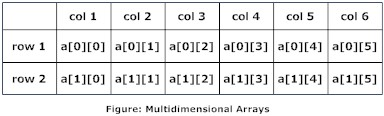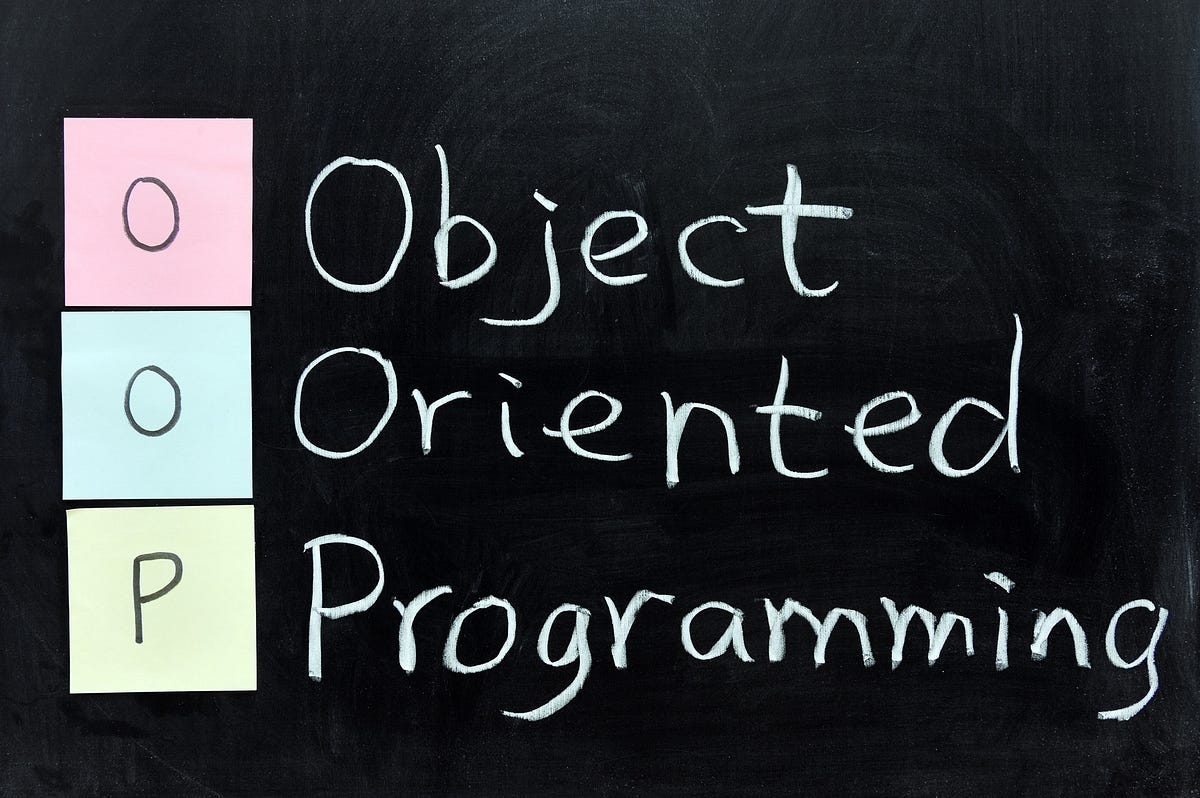# Implementation of 2D Array using 1D array in Object Oriented Programming C++Before Answering the main question it is necessary to discuss some basic concepts; For example, What are array ? and what does the OOP means?

## What are arrays?

In programming, arrays are the sequential data containers of same data type.Here sequential means that arrays occupy the memory addresses in a sequence. Each object in these containers is called element of the array.

Now moving towards the main topic but there’s a question before that Why should we Implement any 2D array using 1D Array? or what’s the benefit of it?

• The very first benefit of it is Efficiency
• Less Time consumption
• Less complex

## What is OOP or Object Oriented Programming ?OOP Object-oriented programming is a programming approach based on the concept of “objects”, which may contain data, in the form of fields, often known as properties; and code, in the form of procedures, often known as methods.

### Implementing the 2d array as 1D array with  code

#include <iostream>
#include <string>

using namespace std;
class DoubleDimensionalArray {
private:
int rows,clms;
int* ptr_arr;
public:

//Creating a array
void createArray(int rows,int clms) {
ptr_arr = new int[rows*clms];
this->rows=rows;
this->clms=clms;

}

//putting an value in the array
void putValue(int rowIndex, int colIndex, int value) {

int si;

//This is the main logic here i am using the row major order here
si=rowIndex*this->clms+colIndex;
ptr_arr[si]=value;
}
void getValue(int rowIndex, int colIndex) {
int si;
si=rowIndex*this->clms+colIndex;
cout<<ptr_arr[si];
}
void search(int value) {
for(int i=0;i<rows*clms;i++) {
if (value==ptr_arr[i]) {
cout << “Your value is found”<<endl;
}
}

}

~DoubleDimensionalArray()
{
delete ptr_arr;
}

};

int main () {

DoubleDimensionalArray v;
v.createArray(1,1);
v.putValue(0,0,3);
v.search(3);

return 0;
}

### Formula of Row Major Order

(RowIndex * No.OfColumns)+ ColumnIndex

### Conclusion

I have added just some functions you can add more if you want. Code is already well commented but if i have missed any important thing then let me know. And ask me if you need any help.

I have also written about Binary Search  which you can also read. If you want suggest any topic then please let me know.

Thanks 😊

1.•